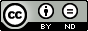### Scalar Singularly Perturbed Cauchy Problem for a Differential Equation of Fractional Order

Burkhan Kalimbetov

#### Abstract

In this paper we consider initial problem for an ordinary differential equation of fractional order with a small parameter for the derivative. S.A. Lomov regularization method is used to construct an asymptotic approximate solution of the problem with accuracy up to any power of a small parameter. Using the computer mathematics system (CMS) Maple, a symbolic solution of the original problem is obtained, and solution schedules are constructed, depending on the initial data and various values of the small parameter. It is shown that the asymptotic solution presented in the form of a specific convergent series and the solution represented by the CMS Maple coincides with the exact solution of the original problem.

#### Keywords

- singular perturbation, asymptotic solution, regularization of the problem, iterative problem, computer mathematics system Maple

PDF

#### References

Khalil, R., Al Horani, M., Yousef, A. and Sababheh, M. (2014) A new definition of fractional derivative, Journal Comput. Appl. Math., 264, 65–70.

Katugampola, U. (2015) Correction to “What is a fractional derivative?” by Ortigueira and Machado. Journal Computational Physics, 293, 4–13.

Lomov, S.A. (1992) Introduction to General Theory of Singular Perturbations, 112, American Mathematical Society, Providence, USA.

Kirsanov, M.N. (2007) Counts of in Maple, M, Fizmatlit.

DOI: https://doi.org/10.24203/ajfam.v7i1.5619

### Refbacks

• There are currently no refbacks.

Copyright (c) 2019 Asian Journal of Fuzzy and Applied Mathematics